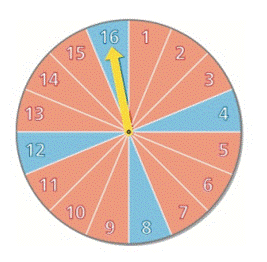Chapter 14.2, Problem 29E### Algebra and Trigonometry (MindTap ...

4th Edition
James Stewart + 2 others
ISBN: 9781305071742

#### Solutions

Chapter
Section### Algebra and Trigonometry (MindTap ...

4th Edition
James Stewart + 2 others
ISBN: 9781305071742
Textbook Problem

# 2 9 - 3 0 Refer to the spinner in Exercises 21 − 22 .Find the probability that the spinner has stopped on an even number, given that it has stopped on red. 2 1 - 2 2 Refer to the spinner shown in the figure. Find the probability of the given event.(a) The spinner stops on red.(b) The spinner stops on an even number.(c) The spinner stops on red or an even number.(a) The spinner stops on blue.(b) The spinner stops on an odd number.(c) The spinner stops on blue or an odd number.

To determine

To find:

The conditional probability that the spinner has stopped on an even number given that it has stopped on red.

Explanation

Given:

The spinner is shown below:

Approach:

p(A|B)=p(AB)p(B)(1)

Here, p(A|B) is the conditional probability of an event A given that B has already occurred, p(AB) is the joint probability of events A and B, p(B) is the probability of event B.

There are 16 numbers in the spinner out of which 8 are even and 8 are odd numbers.

Calculation:

There are 12 red portions and 4 blue portions in the spinner.

Suppose E is the event of even number and R is the event of red portion.

p(E)=816

Here, p(E) is the probability of an even number on the spinner.

p(R)=216

Here, p(R) is the probability of red portion on the spinner.

p(ER)=416

Here, p(ER) is the joint probability of events E and F

### Still sussing out bartleby?

Check out a sample textbook solution.

See a sample solution

#### The Solution to Your Study Problems

Bartleby provides explanations to thousands of textbook problems written by our experts, many with advanced degrees!

Get Started